# COE 561 Digital System Design Synthesis Architectural Synthesis

• Slides: 67
Download presentationCOE 561 Digital System Design & Synthesis Architectural Synthesis Dr. Aiman H. El-Maleh Computer Engineering Department King Fahd University of Petroleum & Minerals [Adapted from slides of Prof. G. De Micheli: Synthesis & Optimization of Digital Circuits]Outline n Motivation n Dataflow graphs & Sequencing graphs n Resources n Synthesis in temporal domain: Scheduling n Synthesis in spatial domain: Binding n Scheduling Models n Algorithmic Solution to the Optimum Binding Problem n Register Binding Problem • Unconstrained scheduling • Scheduling with timing constraints • Scheduling with resource constraints 2Synthesis n Transform behavioral into structural view. n Architectural-level synthesis n Logic-level synthesis • Architectural abstraction level. • Determine macroscopic structure. • Example: major building blocks like adder, register, mux. • Logic abstraction level. • Determine microscopic structure. • Example: logic gate interconnection. 3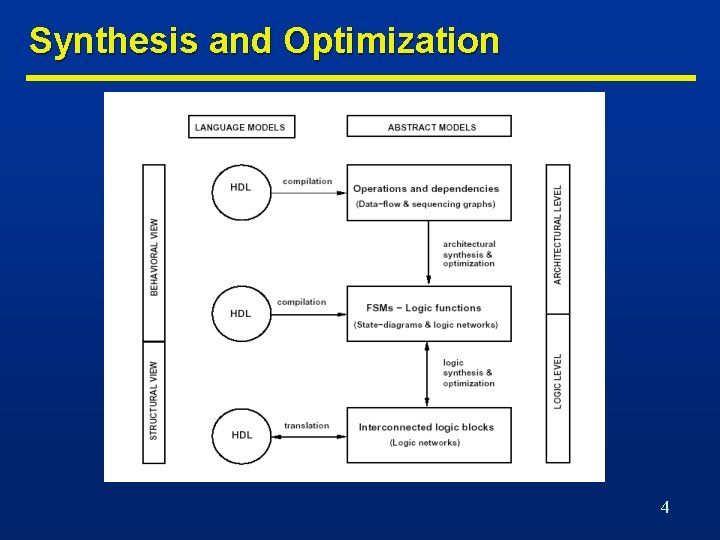Synthesis and Optimization 4Architectural-Level Synthesis Motivation n Raise input abstraction level. n Reduce design time. n Explore and optimize macroscopic structure • Reduce specification of details. • Extend designer base. • Self-documenting design specifications. • Ease modifications and extensions. • Series/parallel execution of operations. 5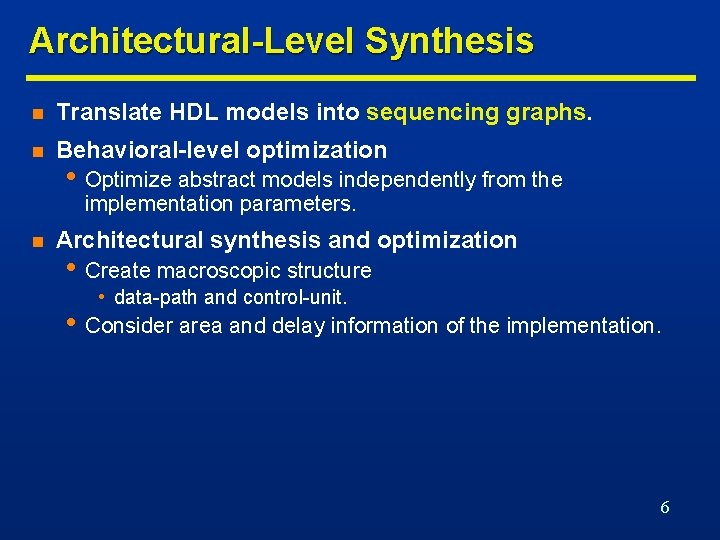Architectural-Level Synthesis n Translate HDL models into sequencing graphs. n Behavioral-level optimization • Optimize abstract models independently from the implementation parameters. n Architectural synthesis and optimization • Create macroscopic structure • data-path and control-unit. • Consider area and delay information of the implementation. 6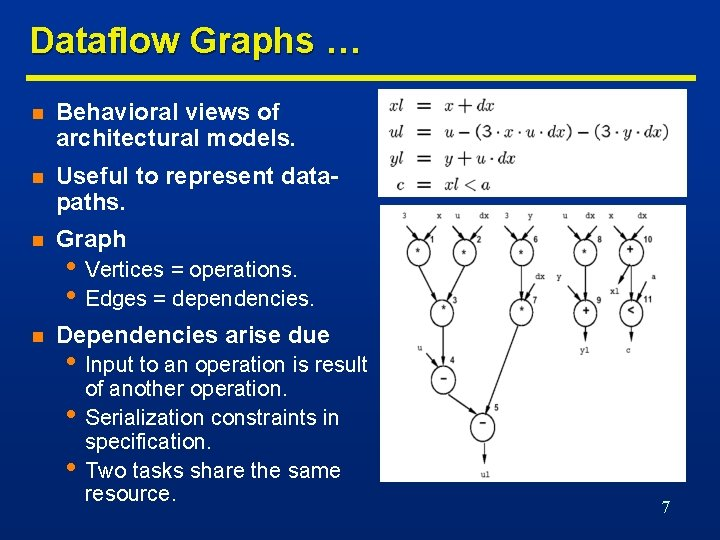Dataflow Graphs … n Behavioral views of architectural models. n Useful to represent datapaths. n Graph n Dependencies arise due • Vertices = operations. • Edges = dependencies. • Input to an operation is result • • of another operation. Serialization constraints in specification. Two tasks share the same resource. 7… Dataflow Graphs n Assumes the existence of variables who store information required and generated by operations. n Each variable has a lifetime which is the interval from birth to death. n Variable birth is the time at which the value is generated. n Variable death is the latest time at which the value is referenced as input to an operation. n Values must be preserved during life-time. 8Sequencing Graphs n Useful to represent data-path and control. n Extended dataflow graphs • Control Data Flow Graphs • • (CDFGs). Polar: source and sink. Operation serialization. Hierarchy. Control-flow commands • branching and iteration. n Paths in the graph represent concurrent streams of operations. 9Behavioral-Level Optimization n Tree-height reduction using commutativity and associativity n x = a + b * c + d => x = (a + d) + b * c n Tree-height reduction using distributivity n x = a * (b * c * d + e) => x=a*b*c*d+a*e 10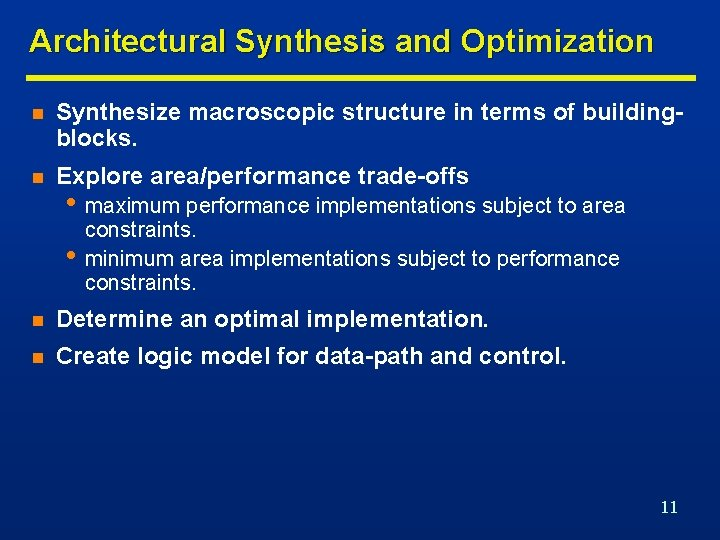Architectural Synthesis and Optimization n Synthesize macroscopic structure in terms of buildingblocks. n Explore area/performance trade-offs • maximum performance implementations subject to area • constraints. minimum area implementations subject to performance constraints. n Determine an optimal implementation. n Create logic model for data-path and control. 11Circuit Specification for Architectural Synthesis n Circuit behavior n Building blocks • Sequencing graphs. • Resources. • Functional resources: process data (e. g. ALU). • Memory resources: store data (e. g. Register). • Interface resources: support data transfer (e. g. MUX and Buses). n Constraints • Interface constraints • Format and timing of I/O data transfers. • Implementation constraints • Timing and resource usage. • Area • Cycle-time and latency 12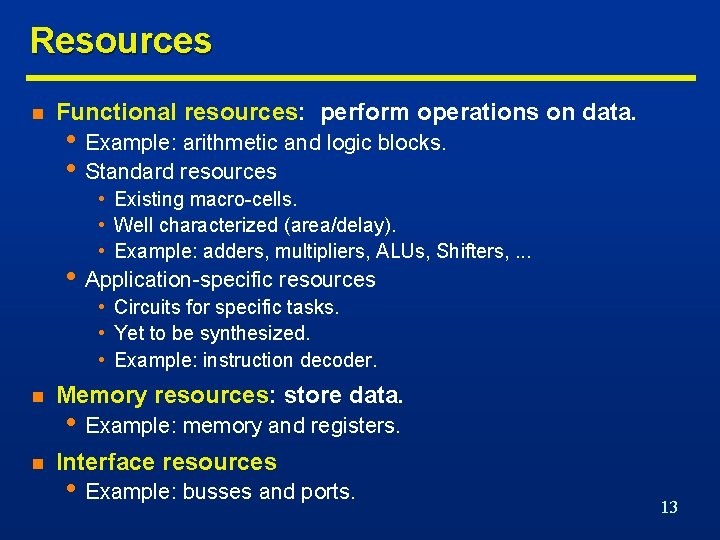Resources n Functional resources: perform operations on data. • Example: arithmetic and logic blocks. • Standard resources • Existing macro-cells. • Well characterized (area/delay). • Example: adders, multipliers, ALUs, Shifters, . . . • Application-specific resources • Circuits for specific tasks. • Yet to be synthesized. • Example: instruction decoder. n Memory resources: store data. n Interface resources • Example: memory and registers. • Example: busses and ports. 13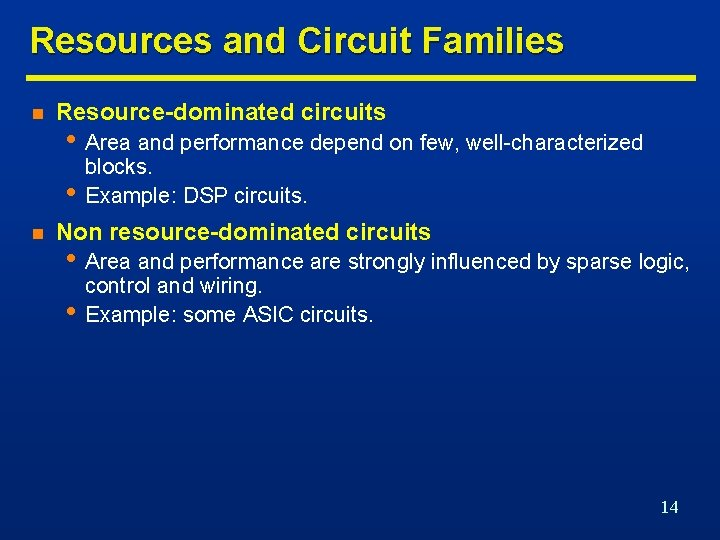Resources and Circuit Families n Resource-dominated circuits • Area and performance depend on few, well-characterized • n blocks. Example: DSP circuits. Non resource-dominated circuits • Area and performance are strongly influenced by sparse logic, • control and wiring. Example: some ASIC circuits. 14Synthesis in the Temporal Domain: Scheduling n Goal n Scheduled sequencing graph n Unconstrained scheduling. n Scheduling with timing constraints. n Scheduling with resource constraints. • Associate a start-time with each operation. • Satisfying all the sequencing (timing and resource) constraints. • Determine area/latency trade-off. • Determine latency and parallelism of the implementation. • Sequencing graph with start-time annotation. 15Scheduling … 4 Multipliers, 2 ALUs 1 Multiplier , 1 ALU 16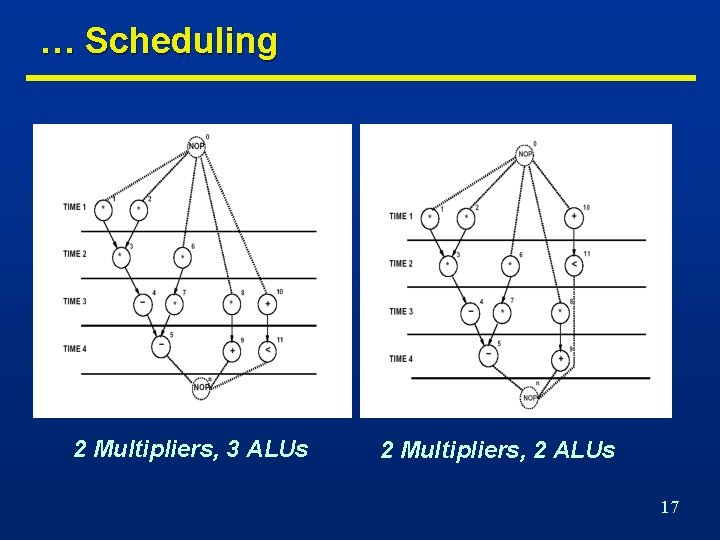… Scheduling 2 Multipliers, 3 ALUs 2 Multipliers, 2 ALUs 17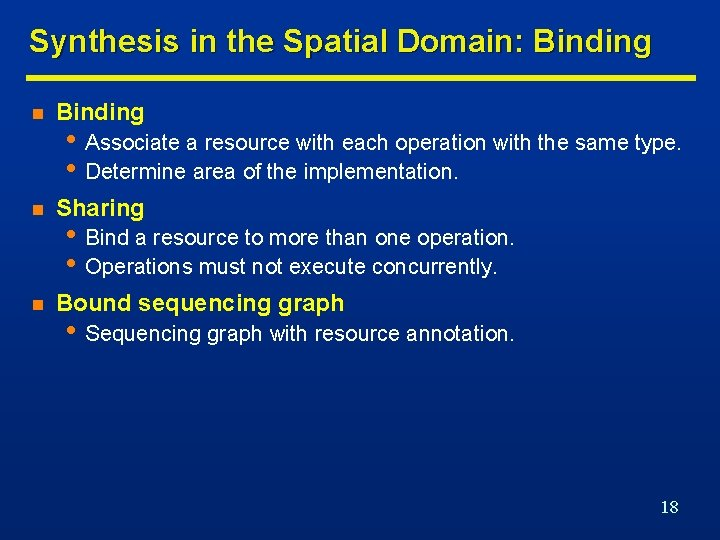Synthesis in the Spatial Domain: Binding n Sharing n Bound sequencing graph • Associate a resource with each operation with the same type. • Determine area of the implementation. • Bind a resource to more than one operation. • Operations must not execute concurrently. • Sequencing graph with resource annotation. 18Example: Bound Sequencing Graph 19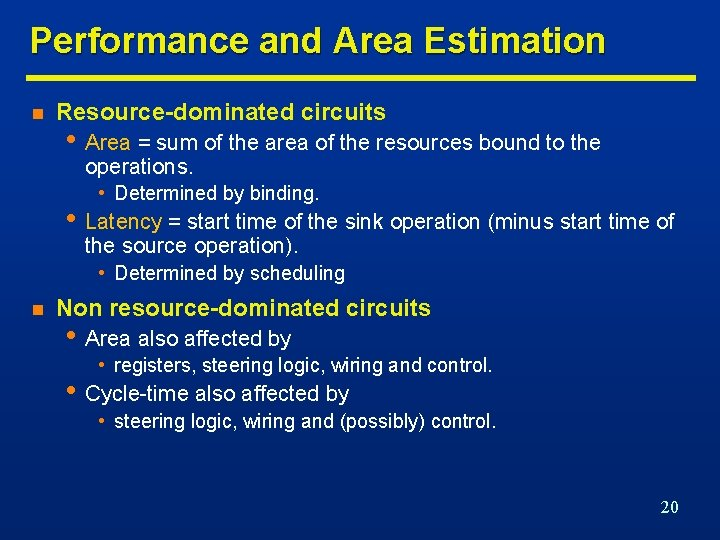Performance and Area Estimation n Resource-dominated circuits • Area = sum of the area of the resources bound to the operations. • Determined by binding. • Latency = start time of the sink operation (minus start time of the source operation). • Determined by scheduling n Non resource-dominated circuits • Area also affected by • registers, steering logic, wiring and control. • Cycle-time also affected by • steering logic, wiring and (possibly) control. 20Scheduling n Circuit model n Scheduling n Goal n Scheduling affects • Sequencing graph. • Cycle-time is given. • Operation delays expressed in cycles. • Determine the start times for the operations. • Satisfying all sequencing (timing and resource) constraints. • Determine area/latency trade-off. • Area: maximum number of concurrent operations of same • type is a lower bound on required hardware resources. Performance: concurrency of resulting implementation. 21Scheduling Models n Unconstrained scheduling. n Scheduling with timing constraints n Scheduling with resource constraints. n Simplest scheduling model • Latency. • Detailed timing constraints. • All operations have bounded delays. • All delays are in cycles. • Cycle-time is given. • No constraints - no bounds on area. • Goal • Minimize latency. 22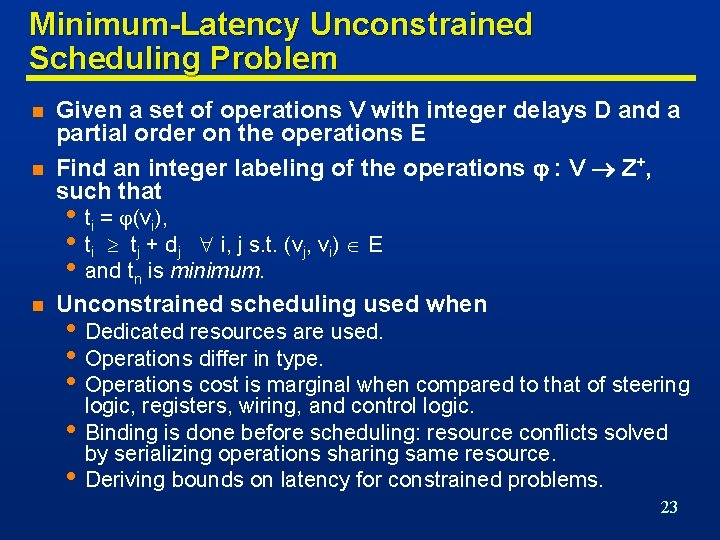Minimum-Latency Unconstrained Scheduling Problem n n Given a set of operations V with integer delays D and a partial order on the operations E Find an integer labeling of the operations : V Z+, such that • ti = (vi), • ti tj + dj i, j s. t. (vj, vi) E • and tn is minimum. n Unconstrained scheduling used when • Dedicated resources are used. • Operations differ in type. • Operations cost is marginal when compared to that of steering logic, registers, wiring, and control logic. • Binding is done before scheduling: resource conflicts solved by serializing operations sharing same resource. • Deriving bounds on latency for constrained problems. 23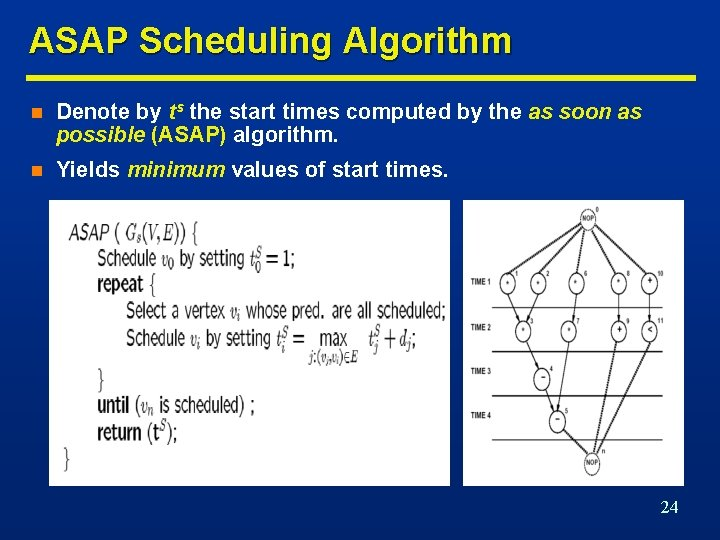ASAP Scheduling Algorithm n Denote by ts the start times computed by the as soon as possible (ASAP) algorithm. n Yields minimum values of start times. 24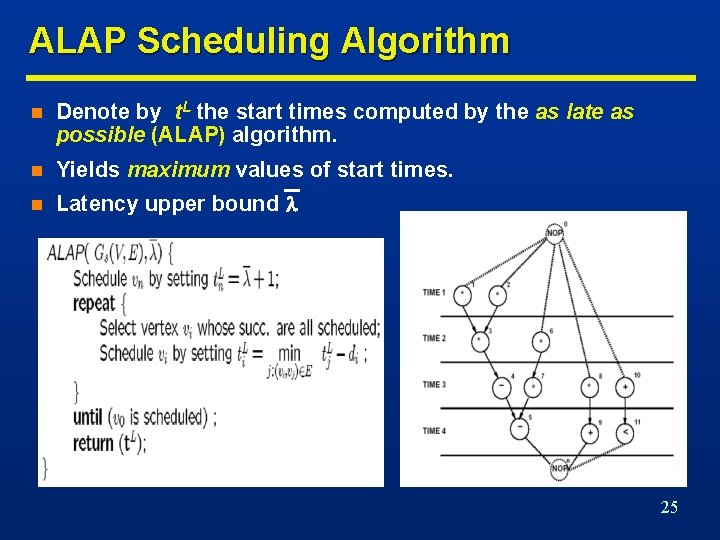ALAP Scheduling Algorithm n Denote by t. L the start times computed by the as late as possible (ALAP) algorithm. n Yields maximum values of start times. n Latency upper bound 25Latency-Constrained Scheduling n ALAP solves a latency-constrained problem. n Latency bound can be set to latency computed by ASAP algorithm. n Mobility • Defined for each operation. • Difference between ALAP and ASAP schedule. • Zero mobility implies that an operation can be started only at • n one given time step. Mobility greater than 0 measures span of time interval in which an operation may start. Slack on the start time. 26Example n n n Operations with zero mobility • • {v 1, v 2, v 3, v 4, v 5}. Critical path. Operations with mobility one • {v 6, v 7}. Operations with mobility two • {v 8, v 9, v 10, v 11} 27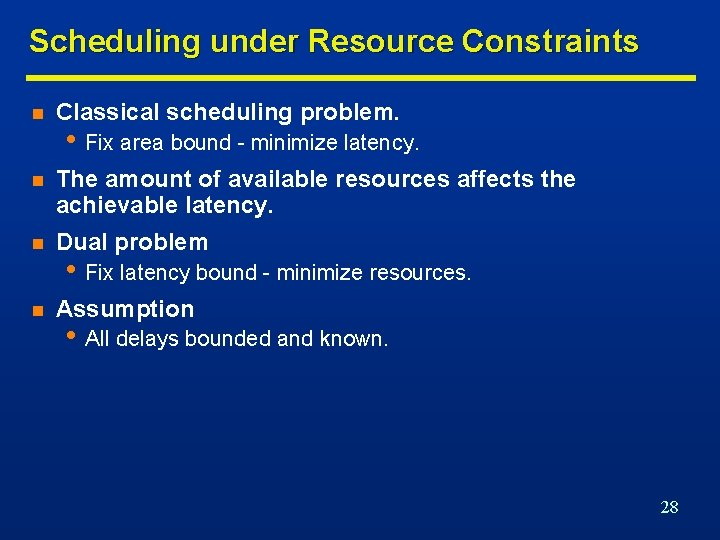Scheduling under Resource Constraints n Classical scheduling problem. n The amount of available resources affects the achievable latency. n Dual problem n Assumption • Fix area bound - minimize latency. • Fix latency bound - minimize resources. • All delays bounded and known. 28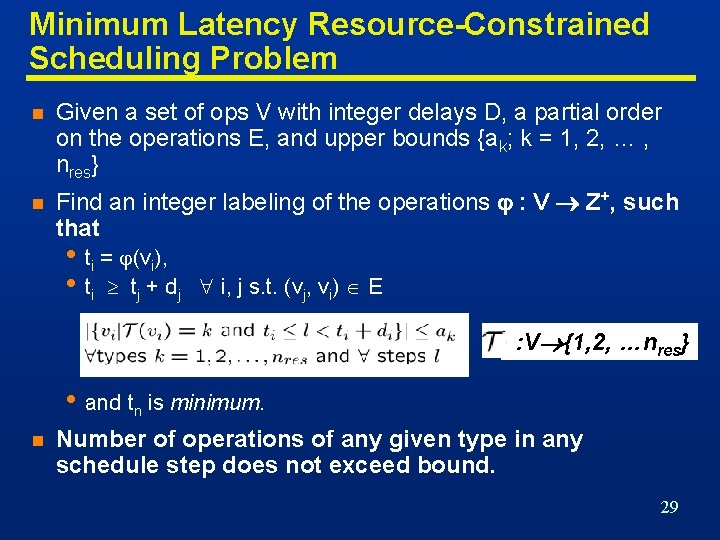Minimum Latency Resource-Constrained Scheduling Problem n Given a set of ops V with integer delays D, a partial order on the operations E, and upper bounds {ak; k = 1, 2, … , nres} n Find an integer labeling of the operations : V Z+, such that • ti = (vi), • ti tj + d j i, j s. t. (vj, vi) E : V {1, 2, …nres} • and tn is minimum. n Number of operations of any given type in any schedule step does not exceed bound. 29Scheduling under Resource Constraints n Intractable problem. n Algorithms • Exact • Integer linear program. • Hu (restrictive assumptions). • Approximate • List scheduling. • Force-directed scheduling. 30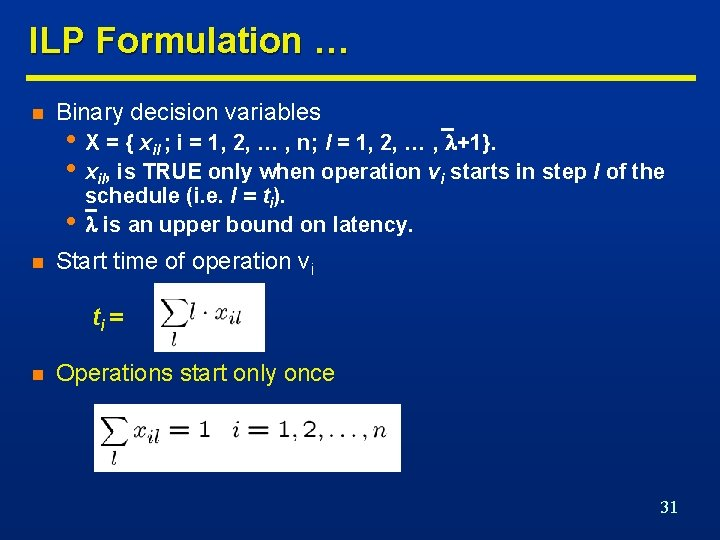ILP Formulation … n Binary decision variables • X = { xil ; i = 1, 2, … , n; l = 1, 2, … , +1}. • xil, is TRUE only when operation vi starts in step l of the • n schedule (i. e. l = ti). is an upper bound on latency. Start time of operation vi ti = n Operations start only once 31… ILP Formulation … n Sequencing relations must be satisfied n Resource bounds must be satisfied 32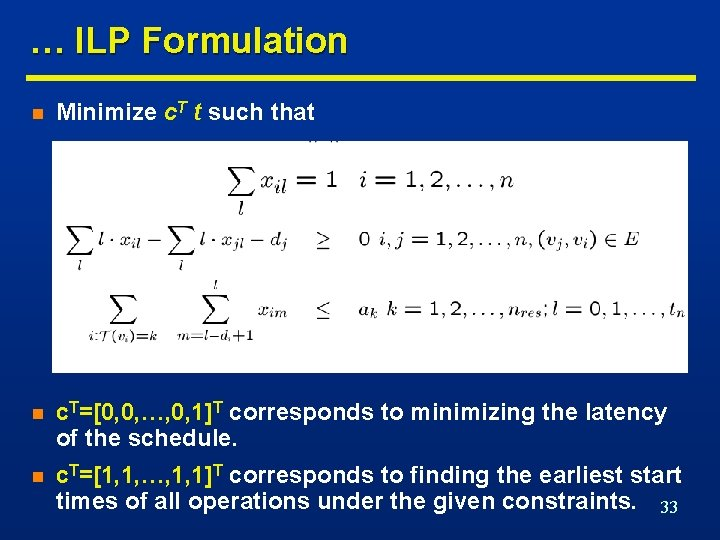… ILP Formulation n Minimize c. T t such that n c. T=[0, 0, …, 0, 1]T corresponds to minimizing the latency of the schedule. n c. T=[1, 1, …, 1, 1]T corresponds to finding the earliest start times of all operations under the given constraints. 33Example … n Resource constraints n Single-cycle operation. n Operations start only once • 2 ALUs; 2 Multipliers. • a 1 = 2; a 2 = 2. • di = 1 i. • x 0, 1=1; x 1, 1=1; x 2, 1=1; x 3, 2=1 • x 4, 3=1; x 5, 4=1 • x 6, 1+ x 6, 2=1 • x 7, 2+ x 7, 3=1 • x 8, 1+ x 8, 2+x 8, 3=1 • x 9, 2+ x 9, 3+x 9, 4=1 • x 10, 1+ x 10, 2+x 10, 3=1 • x 11, 2+ x 11, 3+x 11, 4=1 • xn, 5=1 34… Example … n Sequencing relations must be satisfied • 2 x 3, 2 -x 1, 1 1 • 2 x 3, 2 -x 2, 1 1 • 2 x 7, 2+3 x 7, 3 -x 6, 1 -2 x 6, 2 1 • 2 x 9, 2+3 x 9, 3+4 x 9, 4 -x 8, 1 -2 x 8, 2 -3 x 8, 3 1 • 2 x 11, 2+3 x 11, 3+4 x 11, 4 -x 10, 1 -2 x 10, 2 -3 x 10, 3 1 • 4 x 5, 4 -2 x 7, 2 -3 x 7, 3 1 • 4 x 5, 4 -3 x 4, 3 1 • 5 xn, 5 -2 x 9, 2 -3 x 9, 3 -4 x 9, 4 1 • 5 xn, 5 -2 x 11, 2 -3 x 11, 3 -4 x 11, 4 1 • 5 xn, 5 -4 x 5, 4 1 35… Example n Resource bounds must be satisfied: n Any set of start times satisfying constraints provides a feasible solution. n Any feasible solution is optimum since sink (xn, 5=1) mobility is 0. 36Dual ILP Formulation n Minimize resource usage under latency constraint. n Same constraints as previous formulation. n Additional constraint n Resource usage is unknown in the constraints. n Resource usage is the objective to minimize. • Latency bound must be satisfied. • Minimize c. T a • a vector represents resource usage • c. T vector represents resource costs 37Example n Multiplier area = 5; ALU area = 1. n Objective function: 5 a 1 +a 2. n Start time constraints same. n Sequencing dependency constraints same. n Resource constraints • x 1, 1+x 2, 1+x 6, 1+x 8, 1 – a 1 0 • x 3, 2+x 6, 2+x 7, 2+x 8, 2 – a 1 0 • x 7, 3+x 8, 3 – a 1 0 • x 10, 1 – a 2 0 • x 9, 2+x 10, 2+x 11, 2 – a 2 0 • x 4, 3+x 9, 3+x 10, 3+x 11, 3– a 2 0 • x 5, 4+x 9, 4+x 11, 4– a 2 0 =4 38ILP Solution n Use standard ILP packages. n Transform into LP problem [Gebotys]. n Advantages • Exact method. • Other constraints can be incorporated easily • Maximum and minimum timing constraints n Disadvantages • Works well up to few thousand variables. 39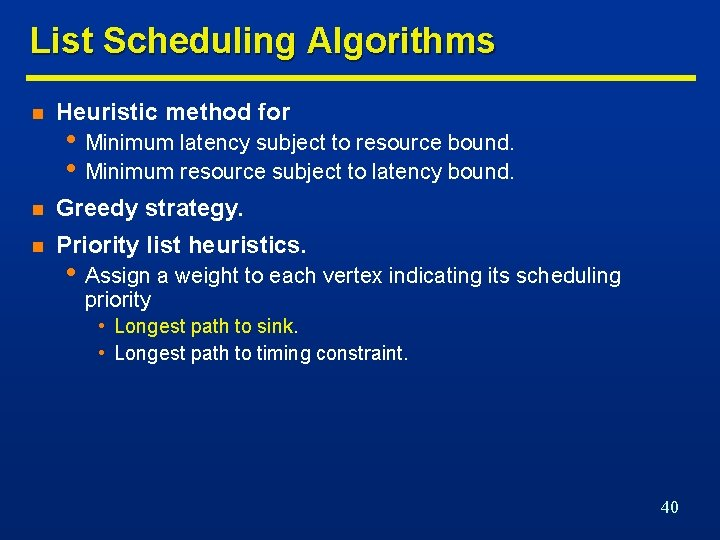List Scheduling Algorithms n Heuristic method for n Greedy strategy. n Priority list heuristics. • Minimum latency subject to resource bound. • Minimum resource subject to latency bound. • Assign a weight to each vertex indicating its scheduling priority • Longest path to sink. • Longest path to timing constraint. 40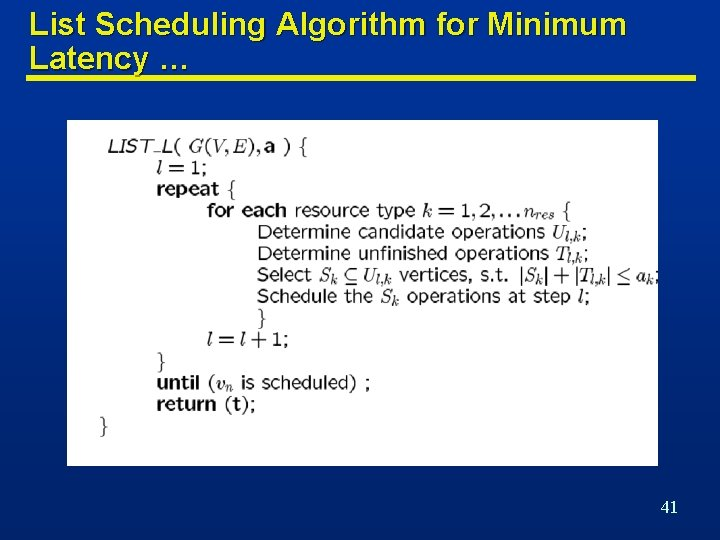List Scheduling Algorithm for Minimum Latency … 41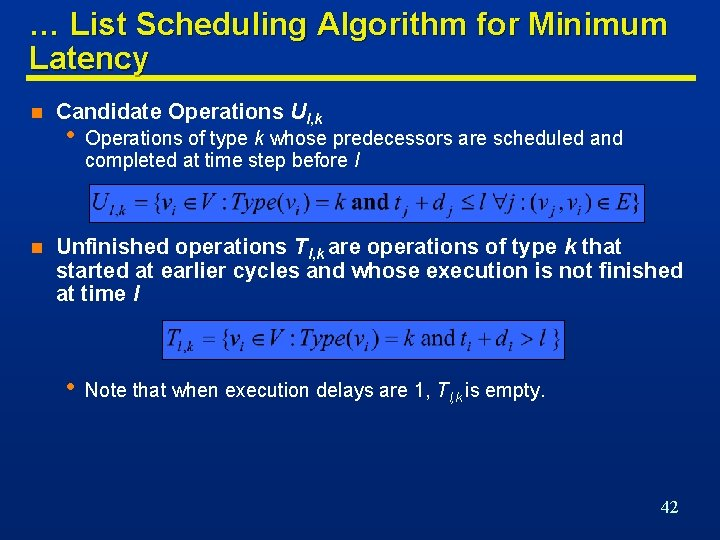… List Scheduling Algorithm for Minimum Latency n n Candidate Operations Ul, k • Operations of type k whose predecessors are scheduled and completed at time step before l Unfinished operations Tl, k are operations of type k that started at earlier cycles and whose execution is not finished at time l • Note that when execution delays are 1, Tl, k is empty. 42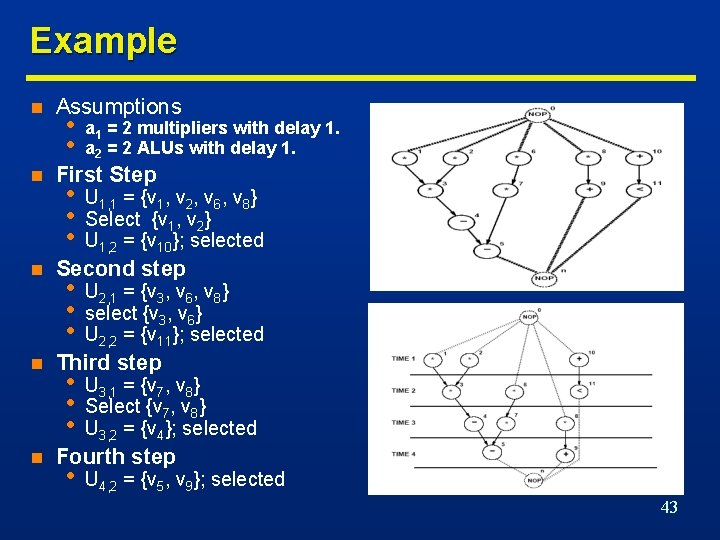Example n n n Assumptions • • a 1 = 2 multipliers with delay 1. a 2 = 2 ALUs with delay 1. First Step • • • U 1, 1 = {v 1, v 2, v 6, v 8} Select {v 1, v 2} U 1, 2 = {v 10}; selected Second step • • • U 2, 1 = {v 3, v 6, v 8} select {v 3, v 6} U 2, 2 = {v 11}; selected Third step • • • U 3, 1 = {v 7, v 8} Select {v 7, v 8} U 3, 2 = {v 4}; selected Fourth step • U 4, 2 = {v 5, v 9}; selected 43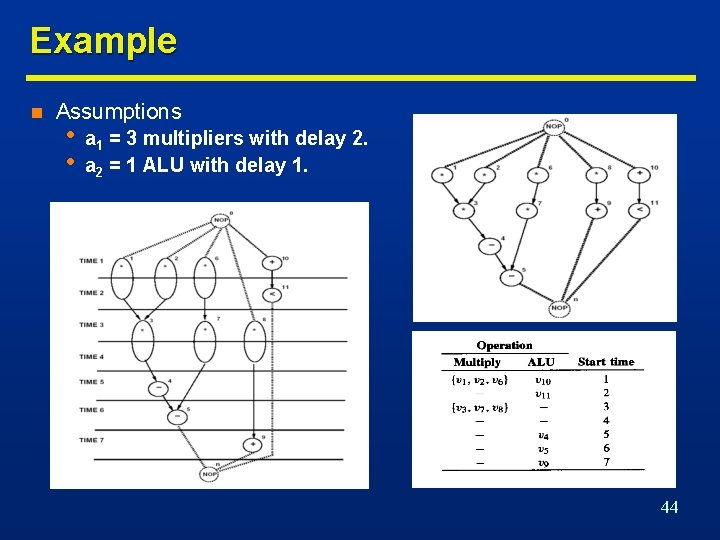Example n Assumptions • • a 1 = 3 multipliers with delay 2. a 2 = 1 ALU with delay 1. 44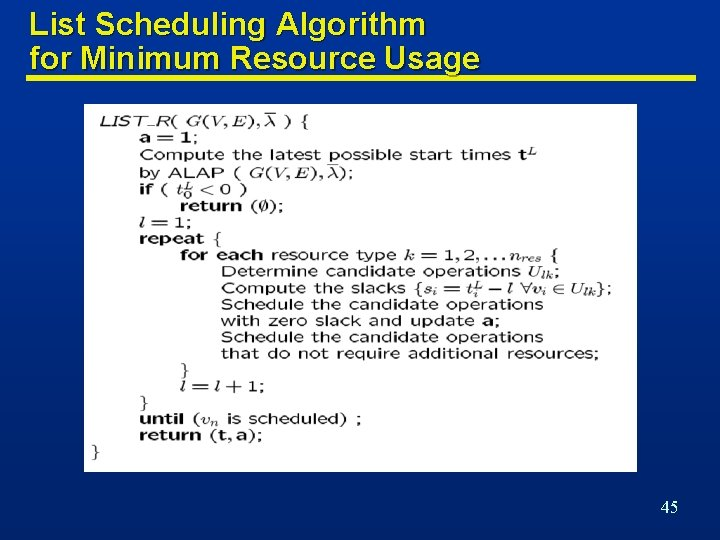List Scheduling Algorithm for Minimum Resource Usage 45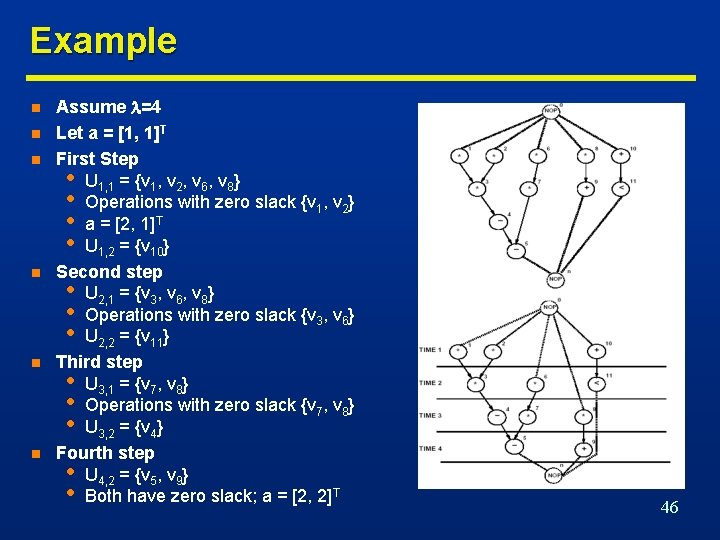Example n n n Assume =4 Let a = [1, 1]T First Step • U 1, 1 = {v 1, v 2, v 6, v 8} • Operations with zero slack {v 1, v 2} • a = [2, 1]T • U 1, 2 = {v 10} Second step • U 2, 1 = {v 3, v 6, v 8} • Operations with zero slack {v 3, v 6} • U 2, 2 = {v 11} Third step • U 3, 1 = {v 7, v 8} • Operations with zero slack {v 7, v 8} • U 3, 2 = {v 4} Fourth step • U 4, 2 = {v 5, v 9} • Both have zero slack; a = [2, 2]T 46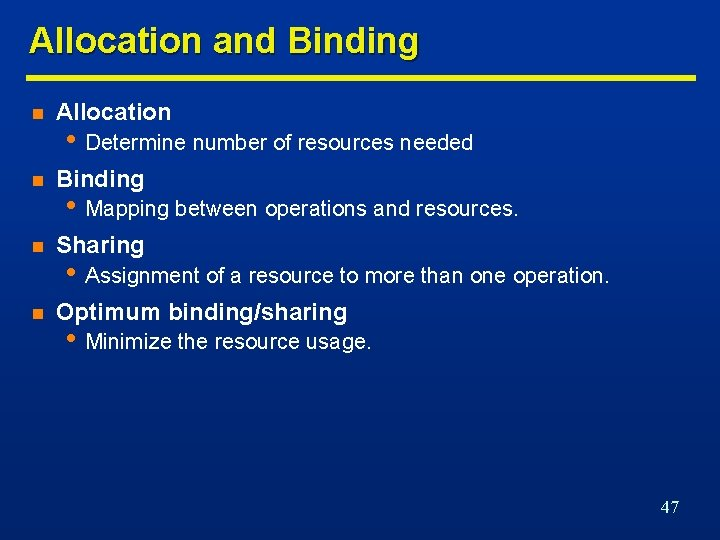Allocation and Binding n Allocation n Binding n Sharing n Optimum binding/sharing • Determine number of resources needed • Mapping between operations and resources. • Assignment of a resource to more than one operation. • Minimize the resource usage. 47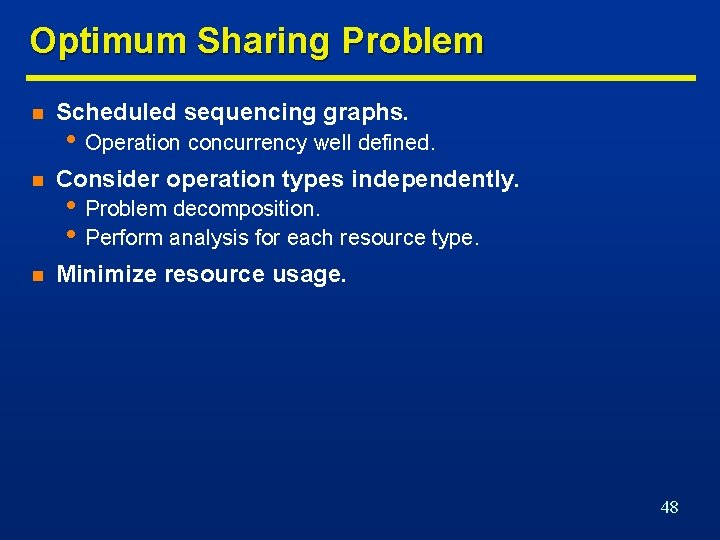Optimum Sharing Problem n Scheduled sequencing graphs. n Consider operation types independently. n Minimize resource usage. • Operation concurrency well defined. • Problem decomposition. • Perform analysis for each resource type. 48Compatibility and Conflicts n n n Operation compatibility • • Same resource type. Non concurrent. Compatibility graph • • Vertices: operations. Edges: compatibility relation. Conflict graph • Complement of compatibility graph. Compatibility graphs Multiplier ALU 49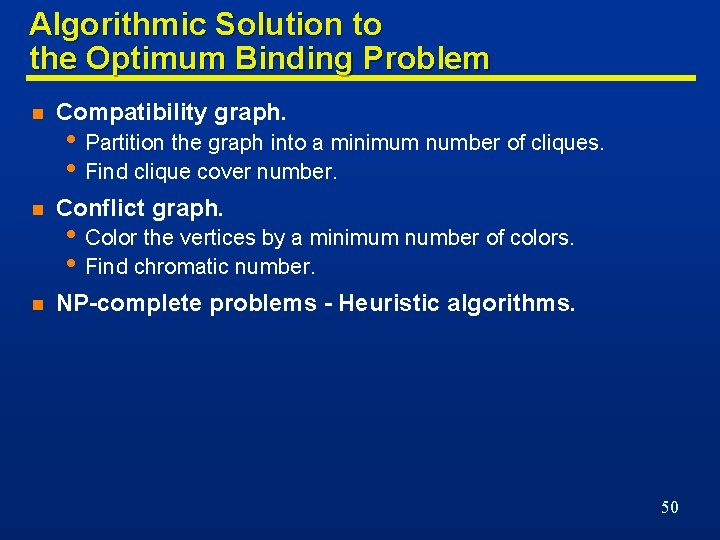Algorithmic Solution to the Optimum Binding Problem n Compatibility graph. n Conflict graph. n NP-complete problems - Heuristic algorithms. • Partition the graph into a minimum number of cliques. • Find clique cover number. • Color the vertices by a minimum number of colors. • Find chromatic number. 50Example 1 2 5 3 2 1 ALU 1: 1, 3, 5 ALU 2: 2, 4 5 4 3 4 51ILP Formulation of Binding n n Boolean variables bir • Operation i bound to resource r. Boolean variables xil • Operation i scheduled to start at step l. n Each operation vi should be assigned to one resource n At most, one operation can be executing, among those assigned to resource r, at any time step 52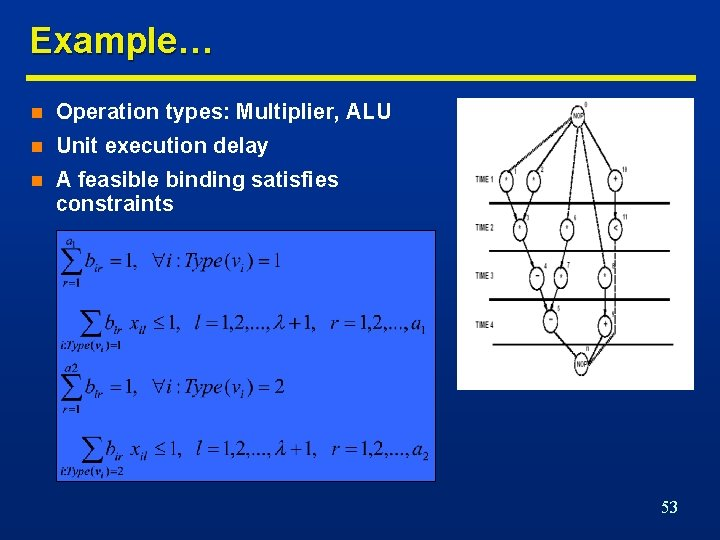Example… n Operation types: Multiplier, ALU n Unit execution delay n A feasible binding satisfies constraints 53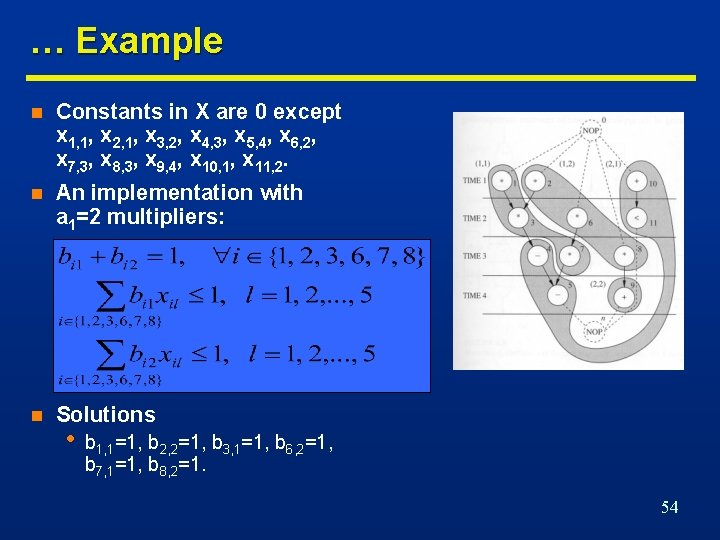… Example n Constants in X are 0 except x 1, 1, x 2, 1, x 3, 2, x 4, 3, x 5, 4, x 6, 2, x 7, 3, x 8, 3, x 9, 4, x 10, 1, x 11, 2. n An implementation with a 1=2 multipliers: n Solutions • b 1, 1=1, b 2, 2=1, b 3, 1=1, b 6, 2=1, b 7, 1=1, b 8, 2=1. 54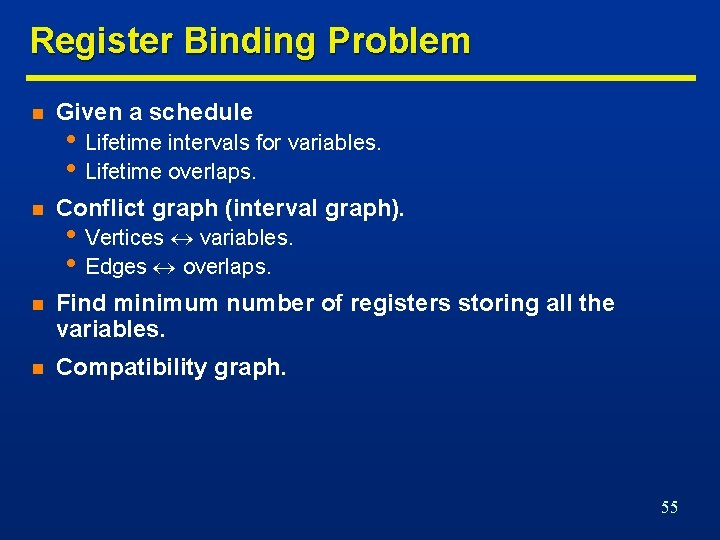Register Binding Problem n Given a schedule n Conflict graph (interval graph). n Find minimum number of registers storing all the variables. n Compatibility graph. • Lifetime intervals for variables. • Lifetime overlaps. • Vertices variables. • Edges overlaps. 55Example n Six intermediate variables that need to be stored in registers {z 1, z 2, z 3, z 4, z 5, z 6} n Six variables can be stored in two registers 56Example n 7 intermediate variables, 3 loop invariants n 5 registers suffice to store 10 intermediate loop variables 57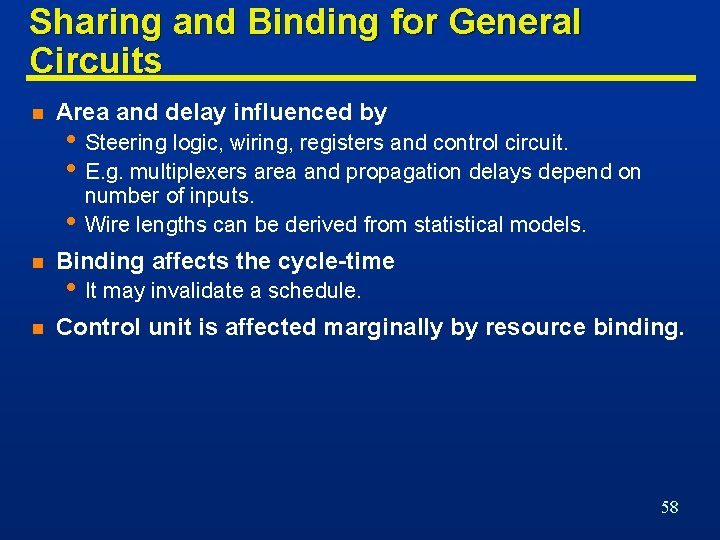Sharing and Binding for General Circuits n Area and delay influenced by • Steering logic, wiring, registers and control circuit. • E. g. multiplexers area and propagation delays depend on • number of inputs. Wire lengths can be derived from statistical models. n Binding affects the cycle-time n Control unit is affected marginally by resource binding. • It may invalidate a schedule. 58Left-Edge Algorithm n n Input • Set of intervals with left and right edge. Rationale • • • Sort intervals by left edge. Assign non-overlapping intervals to first color using the sorted list. When possible intervals are exhausted increase color counter and repeat. 59Example 60Example n It is required to design a circuit to compute the equation Y=A+B+C+D+E+F, where A, B, C, D, E and F are 4 -bit inputs representing unsigned numbers. Assume that inputs are available only during the first cycle when a Start signal is asserted. Assume that a Done signal will be set when the result is ready and the result will remain held until the next Start operation. Assume that the clock cycle delay is constrained by the delay of the adder. n Show a schedule of the operations with minimum latency (i. e. , clock cycles) satisfying the area constraint of using a maximum of two adders. Store the output Y in a register. 61Example 62Example n Show the Data. Path design of your circuit indicating all the control signals and the used adder sizes. 63Example n Show the ASMD diagram of your control unit. 64Example n Consider the network given below with inputs {a, b, c, d} and output {y}: =b+c; =a+b; =+; =b+d; =+; =a+; y=+; Assume that the delay of an Adder fits within one clock cycle. 65Example n Schedule the sequencing graph into the minimum number of cycles under the resource constraints of two Adders 66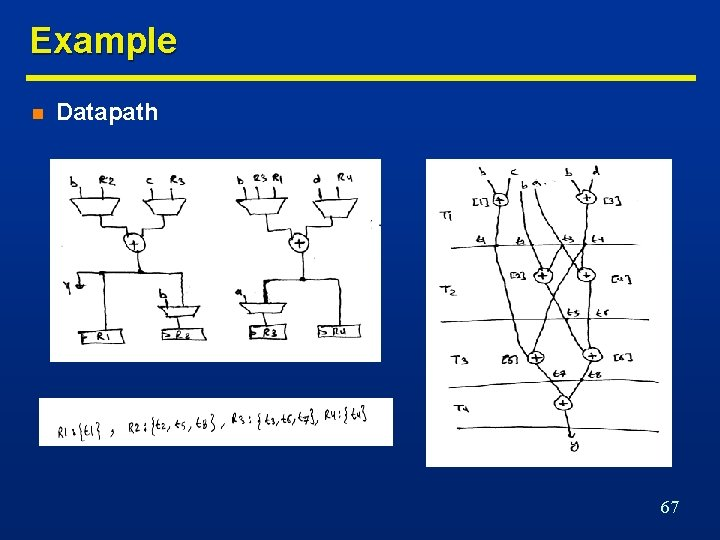Example n Datapath 67